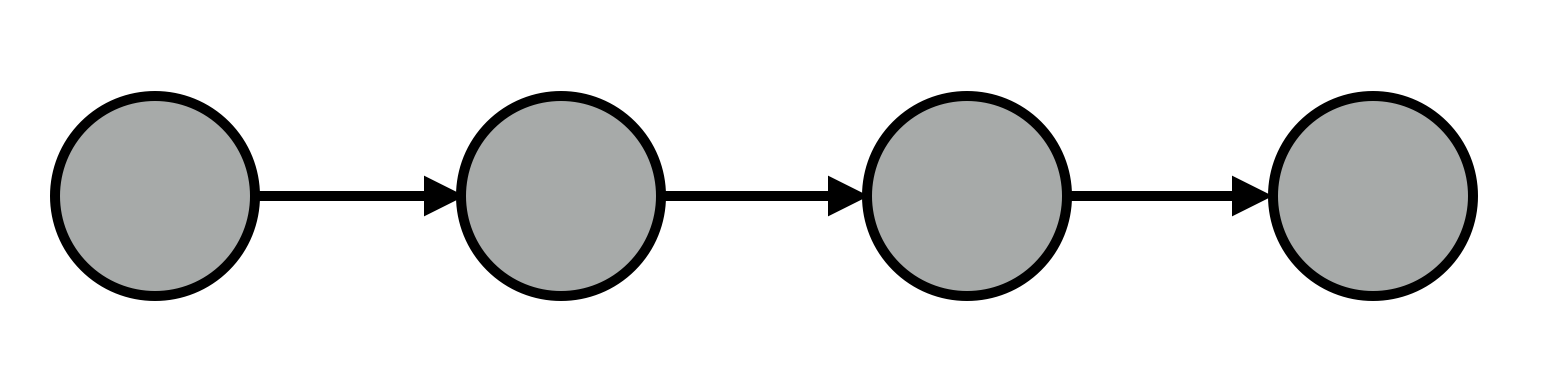Sequences are all over the place. Football games are a sequence of plays, DNA is a sequence of amino acids, and sentences are sequences of words. Having a probabilistic model of how sequences arise can allow you to predict elements that haven’t seen yet. For example:Autocomplete, helping us ask the most important questions first.

Markov models assume that all you need to know to predict an element sequence is which element came before it.

# Sampling from the discrete distribution

Taking a single sample:

``````discrete([1/6, 2/6, 1/6, 1/6, 0.5/6, 0.5/6])
``````

Looking at a histogram of 1000 samples:

``````// look at histogram of 1000 samples
hist(repeat(1000,
function() { discrete([1/6, 2/6, 1/6, 1/6, 0.5/6, 0.5/6]) }))
``````

Using samples from `discrete` to access elements of an array of words:

``````var words = ["^", "complete", "the", "sandwich", "sentence", "\$"];
words[ discrete([1/6, 2/6, 1/6, 1/6, 0.5/6, 0.5/6]) ]
``````

Word salad: building sentences just by `repeat`ing calls to `discrete`.

``````repeat(10,
function() { return words[ discrete([1/6, 2/6, 1/6, 1/6, 0.5/6, 0.5/6]) ] }).join(" ")
``````

These are awful sentences! Note the predominance of the more frequent words.

# Transitioning from one word to another

Defining a transition matrix:

``````var T = {
//                 transition probabilities from current word to
// current word     ^    complete  the   sandwich   sentence    \$
"^":              [ 0,   0.5,      0.3,  0.1,       0.1,        0   ],
"complete":       [ 0,   0,        0.6,  0.2,       0.1,        0.1 ],
"the":            [ 0,   0,        0,    0.6,       0.4,        0   ],
"sandwich":       [ 0,   0,        0,    0,         0,          1   ],
"sentence":       [ 0,   0,        0,    0,         0,          1   ]
};
``````

Defining a function that transitions from one word to the next:

``````var transition = function(word) {
var transitionProbabilities = T[word];
var sampledWordIndex = discrete(transitionProbabilities);
return words[sampledWordIndex];
}
``````

# Recursively transitioning to build up a sentence

``````var _sampleSentence = function(wordsSoFar) {
var prevWord = wordsSoFar[wordsSoFar.length - 1];

if (prevWord == "\$") {
return wordsSoFar;
}

var nextWord = transition(prevWord);

return _sampleSentence( wordsSoFar.concat(nextWord) );
}

var sampleSentence = function() {
return _sampleSentence(["^"]).slice(1,-1).join(" ");
}

hist(repeat(1000, sampleSentence))
``````

# Application: predicting the next part of the sentence (autocomplete)

``````var predictNextPart = function(prefix) {
var words = prefix.split(" ");
var lastWordOfPrefix = _.last(words);
// q: why don't you need to seed the chain with the last word of the prefix?
// why not the *entire* prefix?
return _sampleSentence([lastWordOfPrefix]).slice(1,-1).join(" ");
}

repeat(10, function() { return predictNextPart("complete the") })
``````

# Application: learning the autocomplete transition probabilities from a corpus

for large applications, it’s infeasible to declare the transition matrix by hand. you want to learn it from data.

(we’re using dirichlet as a distribution on distributions)

``````var vocab = "^ \$ factory 500 cookie fortune".split(" ");
var n = vocab.length;

var dirichletParams = repeat(n - 1, function() { return 1 });

var model = function() {

// transition matrix: represented as dictionary
// a key is a single vocab word; its corresponding value
// is an array of transition probabilities
var T = _.object(map(
function(w) {
// don't need to worry about transitions for \$
if (w == "\$") {
return [w, []];
}

// prevent transitions to ^
return [w, .concat(dirichlet(dirichletParams))];

},
vocab));

// given a current word, sample the next word
var transition = function(word) {
var probs = T[word];
return vocab[discrete( probs )];
}

// based on the last entry in a list of words,
// use the transition function to append a next word
var _predict = function(words) {
var prevWord = _.last(words);

if (prevWord == "\$") {
return words;
}

return _predict(words.concat(transition(prevWord)));
}

// convenience method for predicting
var predict = function(string) {
return _predict(("^ " + string).split(" "))
.join(" ")
.replace("^","")
.replace("\$","");
}

var _observe = function(wordsLeft, wordsSoFar) {
var prevWord = _.last(wordsSoFar);
var nextWord = wordsLeft;
if (wordsLeft.length == 0 | prevWord == "\$") {
return wordsSoFar;
}

// sample the next word
var sampledNextWord = transition(prevWord);

factor(sampledNextWord == nextWord ? 0 : -100);

return _observe(wordsLeft.slice(1), wordsSoFar.concat(sampledNextWord));
}

var observe = function(string) {
var words = (string + " \$").split(" ");

return _observe(words, ["^"]) // don't have to observe ^
.join(" ")
.replace("^","")
.replace("\$","");
}